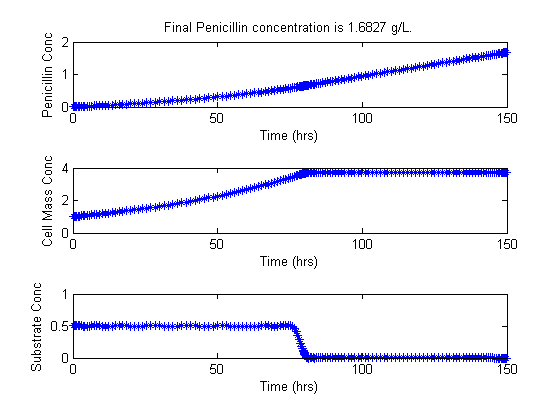86  Penicillin Plant

Fed-batch Fermentor Control: Dynamic Optimization of Batch Processes II. Role of Measurements in Handling Uncertainty 2001, B. Srinivasan, D. Bonvin, E. Visser, S. Palanki

Illustrative example: Nominal Optimization of a Fed-Batch Fermentor for Penicillin Production.

86.1  Problem description

This particular example was featured in the work of B. Srinivasan et al. 2001. The optimal trajectories for the problem was provided in the work.

In this problem, the objective is to maximize the concentration of penicillin, P, produced in a fed-batch bioreactor, given a finite amount of time.

Reactions: S -> X, S -> P
Conditions: Fed-batch, isothermal.
Objective: Maximize the concentration of product P at a given final time.
Manipulated variable: Feed rate of S.
Constraints: Input bounds; upper limit on the biomass concentration,
which is motivated by oxygen-transfer limitation typically occurring at
large biomass concentration.
 J = −P(tf)

subject to:

 X dt
= my*X −
 u V
*X
 S dt
= −
 my*X Yx
−
 v*X Yp
+
 u V
*(SinS
 P dt
= v*X −
 u V
*P
 V dt
= u

my =
 um*S Km+S+S2/Ki

Programmer: Wee Kiat Lim (Nanyang Technological University)

86.2  Problem setup

Penalty for variations in u

penalty_constant = 0.001;

% Various constants
miu_m = 0.02;  Km   = 0.05;  Ki = 5;
Yx    = 0.5;   Yp   = 1.2;   v = 0.004;
Sin   = 200;   umin = 0;     umax = 1;
Xmin  = 0;     Xmax = 3.7;   Smin = 0;

% no. of collocation points to use
narr = [20 80];
for n=narr
toms t1
toms tcut
p1 = tomPhase('p1', t1, 0, tcut, n);

setPhase(p1);
tomStates X1 S1 P1 V1 %Vs %Scaling is disabled here
tomControls u1

% Initial guess
if n == narr(1)
x01 = {tcut == 75
icollocate({X1 == 1+2.7*t1/tcut; S1 == 0.5;
P1 == 0.6*t1/tcut; V1 == 150})
collocate(u1 == 0.03+0.06*t1/tcut)};
else
x01 = {tcut == tcutg
icollocate({X1 == Xg1; S1 == Sg1; P1 == Pg1; V1 == Vg1})
collocate(u1 == ug1)};
end

% Box constraints
cbox1 = {75 <= tcut <= 85
0 <= icollocate(X1) <= Xmax
Smin <= icollocate(S1) <= 100
0 <= icollocate(P1) <= 5
1 <= icollocate(V1) <= 300
umin <= collocate(u1) <= umax};

% Boundary constraints
cbnd1 = initial({X1 == 1; S1 == 0.5;
P1 == 0; V1 == 150});

miu1 = (miu_m*S1)/(Km + S1 + S1^2/Ki);

% ODEs and path constraints
temp11 = miu1*X1;
temp21 = u1/V1;
temp31 = v*X1;
ceq1 = collocate ({
dot(X1) == temp11 - u1/V1*X1
dot(S1) == -temp11/Yx - temp31/Yp + temp21*(Sin - S1)
dot(P1) == temp31 - temp21*P1
dot(V1) == u1});

if n == narr(1)
% No objective in first phase
objective = 0;
else
% Variation penalty
objective = penalty_constant*integrate(dot(u1)^2);
end

toms t2
p2 = tomPhase('p2', t2, tcut, 150-tcut, n);

setPhase(p2);
tomStates X2 S2 P2 V2 %Vs %Scaling is disabled here
tomControls u2

% Initial guess
if n == narr(1)
x02 = {
icollocate({X2 == Xmax; S2 == 0; P2 == 0.6+t2/150; V2 == 150});
collocate(u2 == 0.01);
};
else
x02 = {
icollocate({X2 == Xg2; S2 == Sg2; P2 == Pg2; V2 == Vg2})
collocate(u2 == ug2)
};
end

% Box constraints
umax2 = 0.03;
cbox2 = {0 <= icollocate(X2) <= Xmax
Smin <= icollocate(S2) <= 100
0 <= icollocate(P2) <= 5
1 <= icollocate(V2) <= 300
umin <= collocate(u2) <= umax2
initial(S2) <= 0.2};

miu2 = (miu_m*S2)/(Km + S2 + S2^2/Ki);

% ODEs and path constraints
temp12 = miu2*X2;
temp22 = u2/V2;
temp32 = v*X2;
ceq2 = collocate ({
dot(X2) == temp12 - u2/V2*X2
dot(S2) == -temp12/Yx - temp32/Yp + temp22*(Sin - S2)
dot(P2) == temp32 - temp22*P2
dot(V2) == u2});

initial(S2) == final(p1,S1)
initial(P2) == final(p1,P1)
initial(V2) == final(p1,V1)};

if n == narr(1)
% Objective (Negative sign is added to 'maximize' P)
objective = -final(P2);
ptype = 'lpcon';
solver = 'snopt';
else
objective = objective-final(P2)+penalty_constant*integrate(dot(u2)^2);
ptype = 'con';
solver = 'snopt';
end

% Solve the problem
options = struct;
options.name = 'Penicillin Plant';
Prob = sym2prob(ptype, objective, {cbox1, cbnd1, ceq1, cbox2, ceq2, links}, {x01, x02}, options);
Result = tomRun(solver, Prob, 1);
solution = getSolution(Result);

ug1 = subs(u1,solution);
Xg1 = subs(X1,solution);
Sg1 = subs(S1,solution);
Pg1 = subs(P1,solution);
Vg1 = subs(V1,solution);
ug2 = subs(u2,solution);
Xg2 = subs(X2,solution);
Sg2 = subs(S2,solution);
Pg2 = subs(P2,solution);
Vg2 = subs(V2,solution);
tcutg = solution.tcut;
end
===== * * * =================================================================== * * *
TOMLAB - Tomlab Optimization Inc. Development license  999001. Valid to 2011-02-05
=====================================================================================
Problem: ---  1: Penicillin Plant               f_k      -1.682729746070069400
sum(|constr|)      0.000000953950677808
f(x_k) + sum(|constr|)     -1.682728792119391600
f(x_0)     -1.599999999999999600

Solver: snopt.  EXIT=0.  INFORM=1.
SNOPT 7.2-5 NLP code
Optimality conditions satisfied

FuncEv    1 ConstrEv  950 ConJacEv  950 Iter  282 MinorIter 3095
CPU time: 6.875000 sec. Elapsed time: 7.172000 sec.
===== * * * =================================================================== * * *
TOMLAB - Tomlab Optimization Inc. Development license  999001. Valid to 2011-02-05
=====================================================================================
Problem: ---  1: Penicillin Plant               f_k      -1.682693889838489600
sum(|constr|)      0.000001548138130567
f(x_k) + sum(|constr|)     -1.682692341700359000
f(x_0)     -1.682727190779594400

Solver: snopt.  EXIT=0.  INFORM=1.
SNOPT 7.2-5 NLP code
Optimality conditions satisfied

FuncEv   18 GradEv   16 ConstrEv   16 ConJacEv   16 Iter   15 MinorIter  955
CPU time: 3.656250 sec. Elapsed time: 3.687000 sec.

86.3  Plot result

Optimal states and control trajectories

uopt = subs([collocate(p1,u1);collocate(p2,u2)],solution);
Xopt = subs([collocate(p1,X1);collocate(p2,X2)],solution);
Sopt = subs([collocate(p1,S1);collocate(p2,S2)],solution);
Popt = subs([collocate(p1,P1);collocate(p2,P2)],solution);
Vopt = subs([collocate(p1,V1);collocate(p2,V2)],solution);

t = subs([collocate(p1,t1);collocate(p2,t2)],solution);
np = length(t);

Pfinal=subs(final(p2,P2),solution);

% Plots of the trajectories
figure(1)
subplot(3,1,1);
plot(t,Popt,'*-');
title(['Final Penicillin concentration is ',num2str(Pfinal),' g/L.'])
ylabel('Penicillin Conc')
xlabel('Time (hrs)')
subplot(3,1,2);
plot(t,Xopt,'*-');
ylabel('Cell Mass Conc')
xlabel('Time (hrs)')
subplot(3,1,3);
plot(t,Sopt,'*-');
ylabel('Substrate Conc')
xlabel('Time (hrs)')

figure(2)
subplot(2,1,1);
plot(t,Vopt,'*-');
title(['Final Penicillin concentration is ',num2str(Pfinal),' g/L.'])
ylabel('Volume of medium')
xlabel('Time (hrs)')
subplot(2,1,2);
plot(t,uopt,'*-');
ylabel('Feed flowrate')
xlabel('Time (hrs)')

fprintf('\n')
fprintf('Optimization completed... \n')
fprintf('Final Penicillin concentration is %5.4f g/L.\n',Pfinal)
Optimization completed...
Final Penicillin concentration is 1.6827 g/L.